# Basic embedding with EmbedSOM

## Dataset

We will embed a small dataset created from gaussian clusters positioned in vertices of a 5-dimensional hypercube.

#create the seed dataset
n <- 1024
data <- matrix(c(rep(0,n),rep(1,n)),ncol=1)

for(i in 2:5) data <- cbind(c(rep(0,dim(data)), rep(1, dim(data))),rbind(data,data))

#scatter the points to clusters
set.seed(1)
data <- data + 0.2*rnorm(dim(data)*dim(data))
colnames(data) <- paste0('V',1:5)


This looks relatively nicely from the side (each corner in fact hides 8 separate clusters):

plot(data, pch=19, col=rgb(0,0,0,0.2))

## Warning in plot.xy(xy, type, ...): semi-transparency is not supported on this
## device: reported only once per page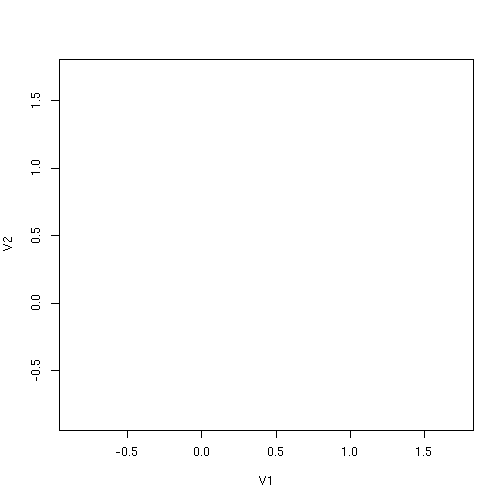Linear dimensionality reduction doesn't help much with seeing all 32 clusters:

plot(data.frame(prcomp(data)$x), pch='.', col=rgb(0,0,0,0.2))  ## Warning in plot.xy(xy.coords(x, y), type = type, ...): semi-transparency is not ## supported on this device: reported only once per page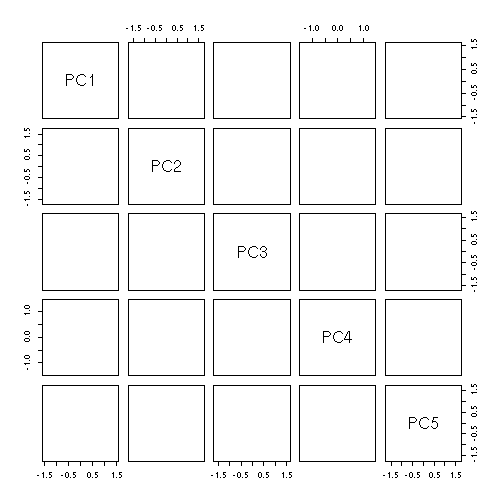Let's use the non-linear EmbedSOM instead. ## Getting the SOM ready EmbedSOM works on a self-organizing map that you need to create first: set.seed(1) map <- EmbedSOM::SOM(data, xdim=24, ydim=24)  EmbedSOM provides some level of compatibility with FlowSOM that can be used to simplify some commands. FlowSOM-originating maps and whole FlowSOM object may be used as well: fs <- FlowSOM::ReadInput(as.matrix(data.frame(data))) fs <- FlowSOM::BuildSOM(fsom=fs, xdim=24, ydim=24)  $$24\times24$$ is the recommended SOM size for getting something interesting from EmbedSOM – it provides a good amount of detail, and still runs quite quickly. ## Embedding When the SOM is ready, a matrix of 2-dimensional coordinates is obtained using the EmbedSOM function: e <- EmbedSOM::EmbedSOM(data=data, map=map)  Alternatively, FlowSOM objects are supported to be used instead of data and map parameters in most EmbedSOM commands: e <- EmbedSOM::EmbedSOM(fsom=fs)  Several extra parameters may be specified; e.g. the following code makes the embedding a bit smoother and faster (but not necessarily better). See the EmbedSOM paper for details on parameters. e <- EmbedSOM::EmbedSOM(data=data, map=map, smooth=2, k=10)  Finally, e now contains the dimensionality-reduced 2D coordinates of the original data that can be used for plotting. head(e)  ## EmbedSOM1 EmbedSOM2 ## [1,] 23.47801 13.42236 ## [2,] 22.86703 12.98544 ## [3,] 23.63919 14.31299 ## [4,] 21.65178 12.51104 ## [5,] 22.58825 13.94369 ## [6,] 23.12144 13.64124  ## Plotting the data The embedding can be plotted using the standard graphics function, nicely showing all clusters next to each other. plot(e, pch=19, cex=.5, col=rgb(0,0,0,0.2))  ## Warning in plot.xy(xy, type, ...): semi-transparency is not supported on this ## device: reported only once per page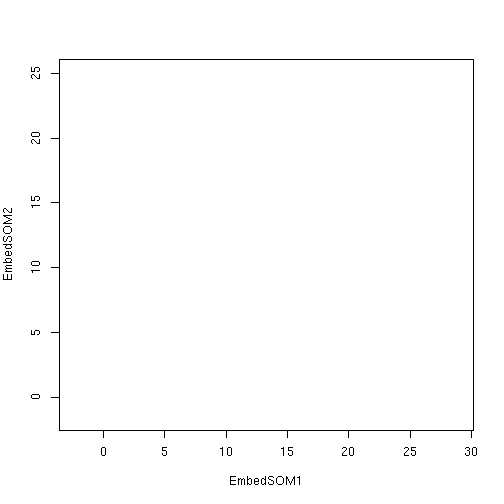EmbedSOM provides specialized plotting function which is useful in many common use cases; for example for displaying density: EmbedSOM::PlotEmbed(e, pch=19, cex=.5, nbin=100)  ## Warning in plot.xy(xy, type, ...): semi-transparency is not supported on this ## device: reported only once per page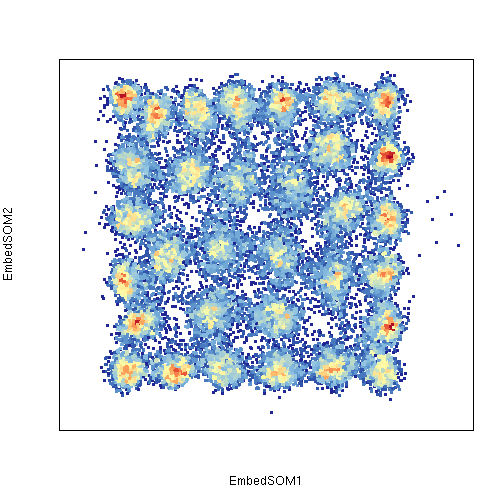Or for seeing colored expression of a single marker (value=1 specifies a column number; column names can be used as well): EmbedSOM::PlotEmbed(e, data=data, pch=19, cex=.5, alpha=0.3, value=1)  ## Warning in plot.xy(xy, type, ...): semi-transparency is not supported on this ## device: reported only once per page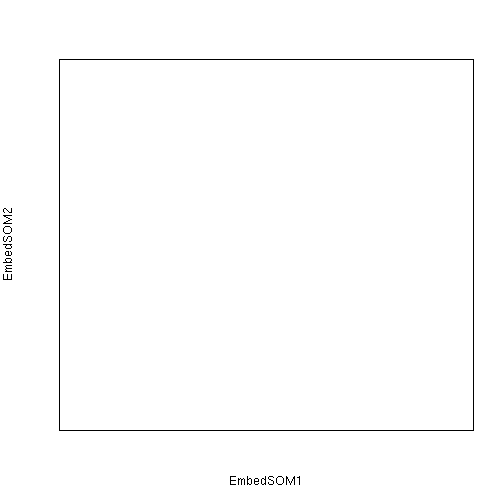(Notice that it is necessary to pass in the original data frame. When working with FlowSOM, the same can be done using fsom=fs.) Or multiple markers: EmbedSOM::PlotEmbed(e, data=data, pch=19, cex=.5, alpha=0.3, red=2, green=4)  ## Warning in plot.xy(xy, type, ...): semi-transparency is not supported on this ## device: reported only once per pageOr perhaps for coloring the clusters. The following example uses the FlowSOM-style clustering to find the original 32 clusters in the scattered data. If that works right, each cluster should have its own color. (See FlowSOM documentation on how the meta-clustering works.) n_clusters <- 32 hcl <- hclust(dist(map$codes))
metaclusters <- cutree(hcl,n_clusters)[map$mapping[,1]] EmbedSOM::PlotEmbed(e, pch=19, cex=.5, clust=metaclusters, alpha=.3)  ## Warning in plot.xy(xy, type, ...): semi-transparency is not supported on this ## device: reported only once per pageCustom colors are also supported (this is colored according to the dendrogram order): colors <- topo.colors(24*24, alpha=.3)[Matrix::invPerm(hcl$order)[map\$mapping[,1]]]

EmbedSOM::PlotEmbed(e, pch=19, cex=.5, col=colors)

## Warning in plot.xy(xy, type, ...): semi-transparency is not supported on this
## device: reported only once per pageggplot2 interoperability is provided using function PlotGG:

EmbedSOM::PlotGG(e, data=data) + ggplot2::geom_hex(bins=80)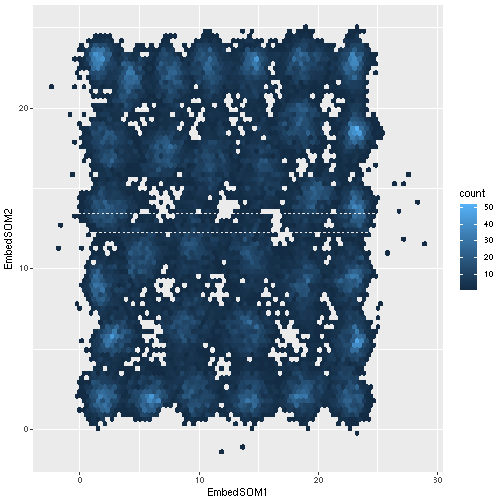(You may also get the ggplot-compatible data object using PlotData function.)